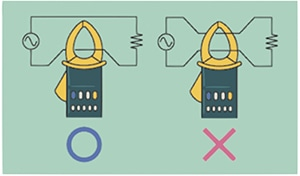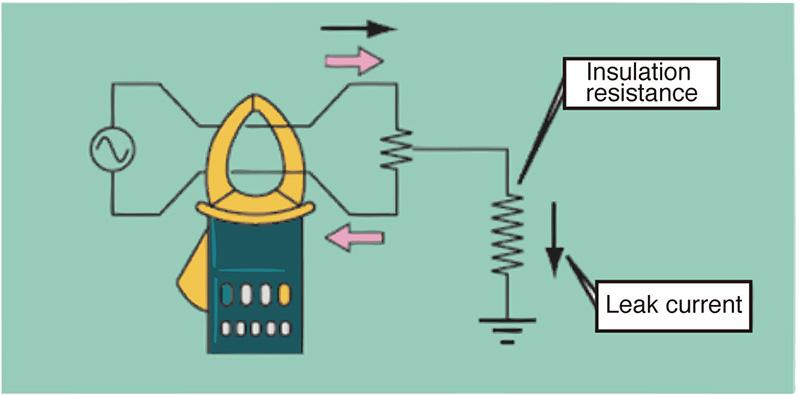# How to use clamp meters

## How to use load current clamp meters

When measuring load current in a 1-phase 2-wire circuit as shown in the figure, the clamp meter should be applied around one (not both) of the wires.

Because the current flowing to and from the load in the two wires forms a pair of magnetic fields that are opposite one another, the two magnetic fields cancel each other out, causing the clamp meter to indicate a current value of zero if two wires are measured at the same time.

When measuring a 3-phase circuit, it is necessary to measure the current value in each of the R, S, and T wires by applying the clamp meter to each in turn.## How to use leakage current clamp meters

When measuring leakage current in a 1-phase/2-wire circuit as shown in the figure, the clamp meter should be applied around both wires.

Because the current flowing to and from the load in the two wires forms a pair of magnetic fields that are opposite one another, there will be a difference in the two fields if there is any leakage current. The measured current value when the clamp meter is applied around both wires at the same time is the leakage current.

When measuring a 3-phase circuit, the clamp meter should be applied around the R, S, and T wires (all three wires) at once. Leakage current can also be measured by applying the clamp meter around a grounding wire.

•